# ADA1: Class 18, Hypothesis testing (one- and two-sample)

Advanced Data Analysis 1, Stat 427/527, Fall 2022, Prof. Erik Erhardt, UNM

Author

Published

August 13, 2022

Include your answers in this document in the sections below the rubric.

# Rubric

Answer the questions with the two data examples.

# Mechanics of a hypothesis test (review)

1. Set up the null and alternative hypotheses in words and notation.

• In words: The population mean for [what is being studied] is different from [value of $$\mu_0$$].’’ (Note that the statement in words is in terms of the alternative hypothesis.)
• In notation: $$H_0: \mu=\mu_0$$ versus $$H_A: \mu \ne \mu_0$$ (where $$\mu_0$$ is specified by the context of the problem).
2. Choose the significance level of the test, such as $$\alpha=0.05$$.

3. Compute the test statistic, such as $$t_{s} = \frac{\bar{Y}-\mu_0}{SE_{\bar{Y}}}$$, where $$SE_{\bar{Y}}=s/\sqrt{n}$$ is the standard error.

4. Determine the tail(s) of the sampling distribution where the $$p$$-value from the test statistic will be calculated (for example, both tails, right tail, or left tail). (Historically, we would compare the observed test statistic, $$t_{s}$$, with the critical value $$t_{\textrm{crit}}=t_{\alpha/2}$$ in the direction of the alternative hypothesis from the $$t$$-distribution table with degrees of freedom $$df = n-1$$.)

5. State the conclusion in terms of the problem.

• Reject $$H_0$$ in favor of $$H_A$$ if $$p\textrm{-value} < \alpha$$.
• Fail to reject $$H_0$$ if $$p\textrm{-value} \ge \alpha$$. (Note: We DO NOT accept $$H_0$$.)
6. Check assumptions of the test (next week).

# Height data for our class

Is the population mean height of UNM students eligible to take Stat 427/527 different from the US average for men (5 ft 9 1/2 in) or women (5 ft 4 in)?

library(erikmisc)
── Attaching packages ─────────────────────────────────────── erikmisc 0.1.16 ──
✔ tibble 3.1.8     ✔ dplyr  1.0.9
── Conflicts ─────────────────────────────────────────── erikmisc_conflicts() ──
✖ dplyr::lag()    masks stats::lag()
erikmisc, solving common complex data analysis workflows
by Dr. Erik Barry Erhardt <erik@StatAcumen.com>
library(tidyverse)
── Attaching packages
───────────────────────────────────────
tidyverse 1.3.2 ──
✔ ggplot2 3.3.6     ✔ purrr   0.3.4
✔ tidyr   1.2.0     ✔ stringr 1.4.0
✔ readr   2.1.2     ✔ forcats 0.5.1
── Conflicts ────────────────────────────────────────── tidyverse_conflicts() ──
✖ dplyr::lag()    masks stats::lag()
# Height vs Hand Span
dat_hand <-
na.omit() %>%
mutate(
Gender_M_F = factor(Gender_M_F, levels = c("F", "M"))
)
Rows: 378 Columns: 6
── Column specification ────────────────────────────────────────────────────────
Delimiter: ","
chr (2): Semester, Gender_M_F
dbl (4): Table, Person, Height_in, HandSpan_cm

ℹ Use spec() to retrieve the full column specification for this data.
ℹ Specify the column types or set show_col_types = FALSE to quiet this message.
str(dat_hand)
tibble [237 × 6] (S3: tbl_df/tbl/data.frame)
$Semester : chr [1:237] "F15" "F15" "F15" "F15" ...$ Table      : num [1:237] 1 1 1 1 1 1 1 1 2 2 ...
$Person : num [1:237] 1 2 3 4 5 6 7 8 1 2 ...$ Gender_M_F : Factor w/ 2 levels "F","M": 2 1 1 1 2 2 1 2 2 1 ...
$Height_in : num [1:237] 69 66 65 62 67 67 65 70 67 63 ...$ HandSpan_cm: num [1:237] 21.5 20 20 18 19.8 23 22 21 21.2 16.5 ...
- attr(*, "na.action")= 'omit' Named int [1:141] 9 13 14 15 16 17 18 22 23 24 ...
..- attr(*, "names")= chr [1:141] "9" "13" "14" "15" ...

Plot the estimated mean from our class sample versus the true US mean.

## If we create a summary data.frame with a similar structure as our data, then we
##   can annotate our plot with those summaries.

est_mean <-
dat_hand %>%
group_by(
Gender_M_F
) %>%
summarize(
Height_in = mean(Height_in)
, .groups = "drop_last"
) %>%
ungroup() %>%
mutate(
TrueEst = "Est"
)

true_mean <-
tribble(
~Gender_M_F, ~Height_in, ~TrueEst
,         "F",       64.0,   "True"
,         "M",       69.5,   "True"
)

trueest_mean <-
est_mean %>%
bind_rows(
true_mean
)

trueest_mean
# A tibble: 4 × 3
Gender_M_F Height_in TrueEst
<chr>          <dbl> <chr>
1 F               65.2 Est
2 M               70.3 Est
3 F               64   True
4 M               69.5 True   

Here’s two ways to plot our data, annotating the observed and hypothesized means.

library(ggplot2)
p1 <- ggplot(data = dat_hand, aes(x = Gender_M_F, y = Height_in))
p1 <- p1 + geom_boxplot(alpha = 1/4)
p1 <- p1 + geom_jitter(position = position_jitter(width = 0.1))
p1 <- p1 + geom_point(data = trueest_mean, aes(colour = TrueEst, shape = TrueEst), size = 4, alpha = 3/4)
p1 <- p1 + labs(title = "Boxplots")
#print(p1)

library(ggplot2)
p2 <- ggplot(data = dat_hand, aes(x = Height_in))
p2 <- p2 + geom_histogram(binwidth = 1)
p2 <- p2 + geom_vline(data = trueest_mean, aes(xintercept = Height_in, colour = TrueEst, linetype = TrueEst))
p2 <- p2 + facet_grid(Gender_M_F ~ .)
p2 <- p2 + labs(title = "Histograms")
#print(p2)

# grid.arrange() is a way to arrange several ggplot objects
library(grid)
library(gridExtra)

Attaching package: 'gridExtra'
The following object is masked from 'package:dplyr':

combine
  lay <-
rbind(
c(1, 2, 2)  # let Plot 2 take twice as much horizontal space as Plot 1
)
grid.arrange(
grobs = list(p1, p2)
, layout_matrix = lay
, top = "Two ways to display the data"
)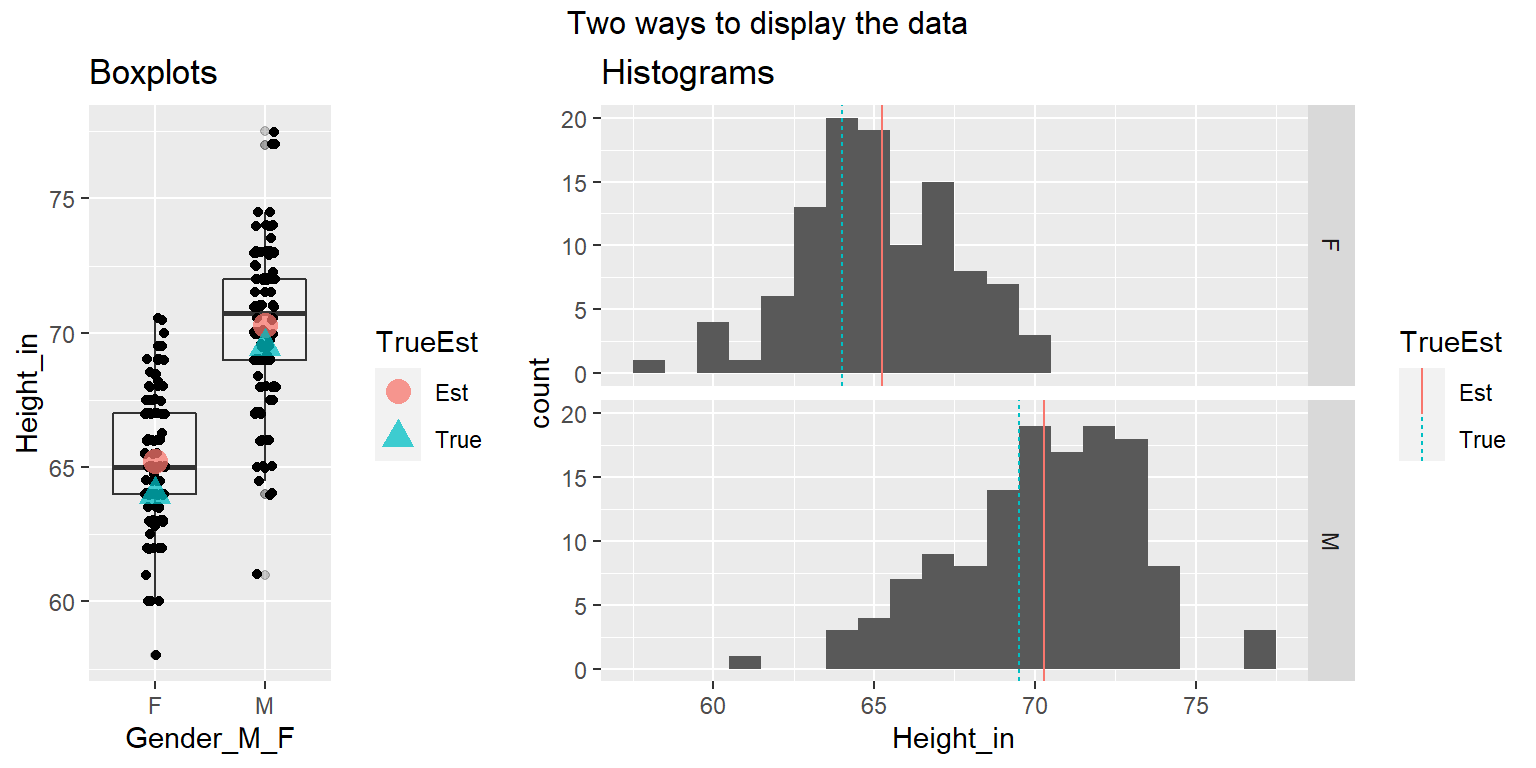## Conduct the hypothesis tests (example)

# look at help for t.test
# ?t.test
# defaults include: alternative = "two.sided", conf.level = 0.95

### Model example: Test female height equal to US.

# test females
t_summary_F <-
t.test(
dat_hand %>% filter(Gender_M_F == "F") %>% pull(Height_in)
, mu = 64
, alternative = "two.sided"
)

t_summary_F

One Sample t-test

data:  dat_hand %>% filter(Gender_M_F == "F") %>% pull(Height_in)
t = 5.2102, df = 106, p-value = 9.351e-07
alternative hypothesis: true mean is not equal to 64
95 percent confidence interval:
64.76972 65.71533
sample estimates:
mean of x
65.24252 
names(t_summary_F)
  "statistic"   "parameter"   "p.value"     "conf.int"    "estimate"
 "null.value"  "stderr"      "alternative" "method"      "data.name"  
e_plot_ttest_pval(t_summary_F)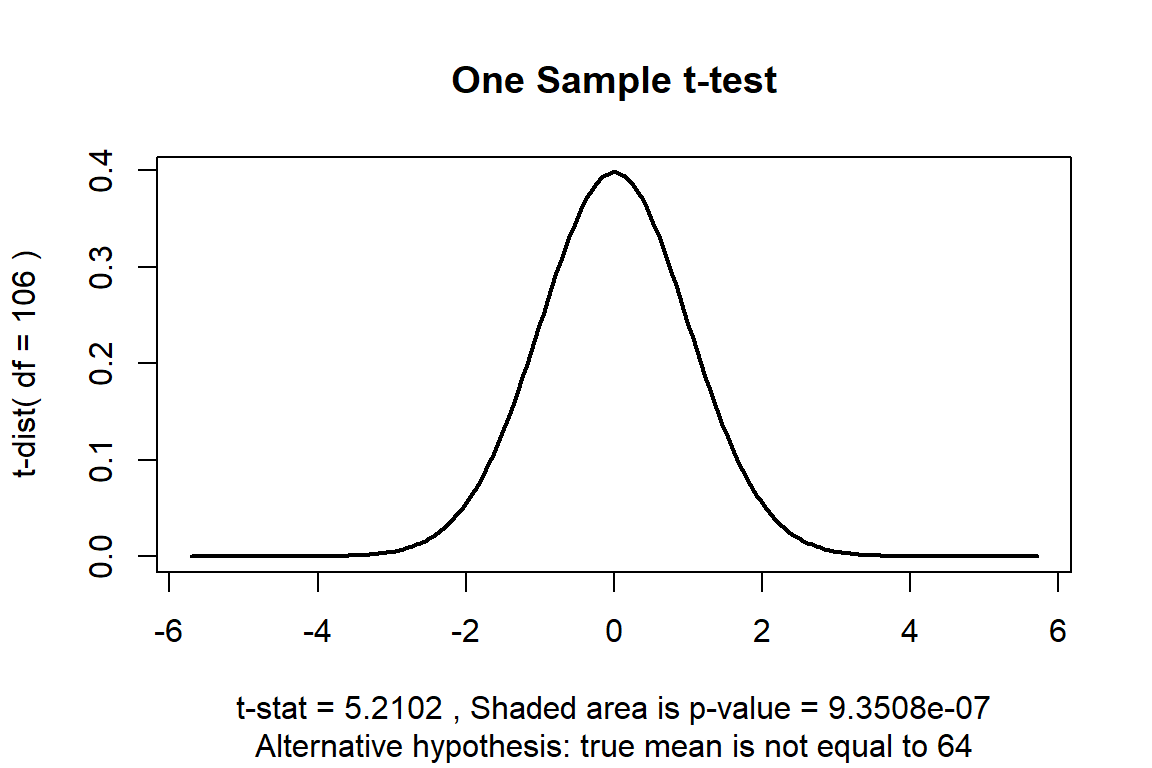Hypothesis test

1. The population mean height for females at UNM eligible to take Stat 427/527 is different from the US population value of $$\mu_0=64$$ inches.’’

• $$H_0: \mu = 64$$ versus $$H_A: \mu \ne 64$$
2. Let $$\alpha = 0.05$$, the significance level of the test and the Type-I error probability if the null hypothesis is true.

3. $$t_{s} = 5.21$$.

4. $$p = 9.35\times 10^{-7}$$, this is the observed significance of the test.

5. Because $$p = 9.35\times 10^{-7} < 0.05$$, we have sufficient evidence to reject $$H_0$$, concluding that the observed mean height is different than the US population mean.

(3 p) As above, set up the hypothesis test for males, but whether UNM males are taller on average than males in the US population.

### Your turn: Test male height greater than US.

## You'll need to modify the statement below to correspond
## to the hypothesis you wish to test

# test males
t_summary_M <-
t.test(
dat_hand %>% filter(Gender_M_F == "M") %>% pull(Height_in)
, mu = 0
, alternative = "two.sided"
)

t_summary_M

One Sample t-test

data:  dat_hand %>% filter(Gender_M_F == "M") %>% pull(Height_in)
t = 279.52, df = 129, p-value < 2.2e-16
alternative hypothesis: true mean is not equal to 0
95 percent confidence interval:
69.79972 70.79490
sample estimates:
mean of x
70.29731 
e_plot_ttest_pval(t_summary_M)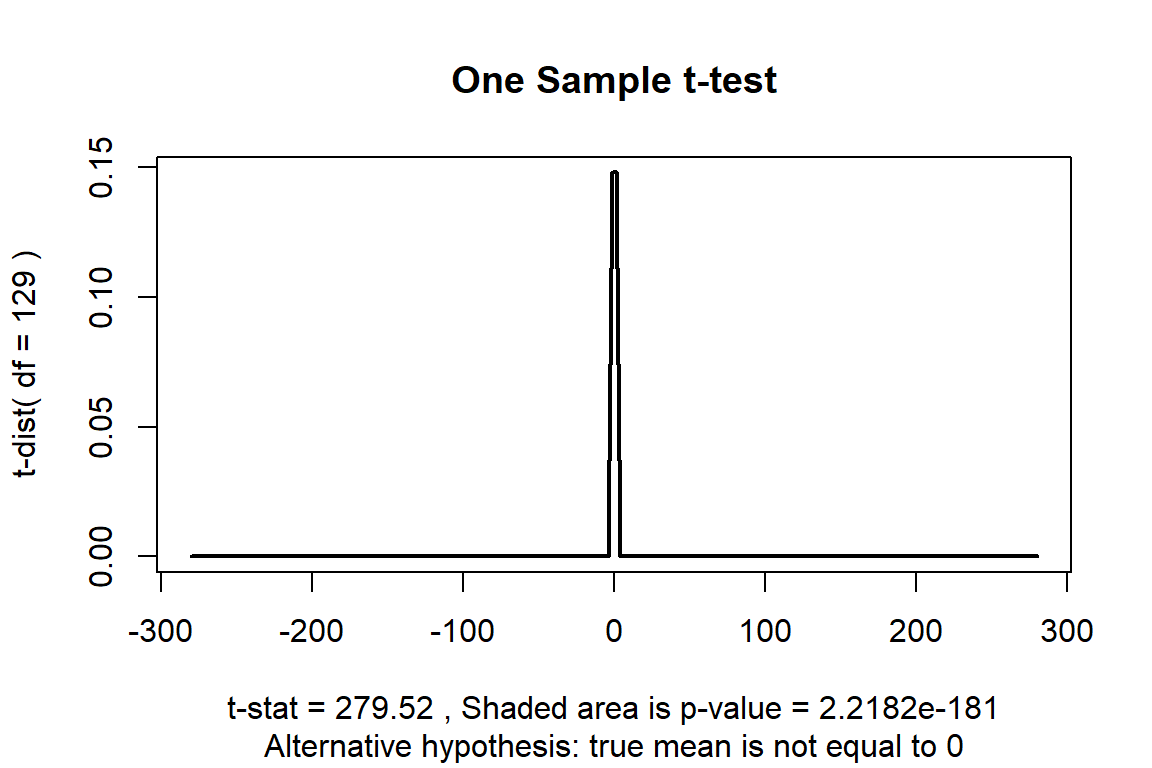Hypothesis test

1. ’’

• $H_0:$ versus $H_A:$
2. Let $$\alpha=0.05$$, the significance level of the test and the Type-I error probability if the null hypothesis is true.

3. $t_{s} =$.

4. $p =$, this is the observed significance of the test.

5. Because $p =$, …

• Also, given your conclusion, state whether you could have made a Type-I or Type-II error and why it is one but not the other.

# Earth’s water example

(3 p) In class we sampled the beach ball and observed 21 of 35 observations were water. Conduct a hypothesis test to determine whether the proportion of water on the beach ball is different from the amount of water on the earth’s surface (71%).

## notes for prop.test() and binom.test()
# x = number of "successes"
# n = total sample size

#n = 2
#x = 1
x = 21
n = 21 + 14

dat_globe <-
tribble(
~type   , ~freq , ~prop
, "Water" ,     x ,      x  / n
, "Land"  , n - x , (n - x) / n
)

dat_globe
# A tibble: 2 × 3
type   freq  prop
<chr> <dbl> <dbl>
1 Water    21   0.6
2 Land     14   0.4
# # prop.test() is an asymptotic (approximate) test for a binomial random variable
# p_summary <- prop.test(x = x, n = n, conf.level = 0.95)
# p_summary

# binom.test() is an exact test for a binomial random variable
b_summary <- binom.test(x = x, n = n, conf.level = 0.95)

b_summary

Exact binomial test

data:  x and n
number of successes = 21, number of trials = 35, p-value = 0.3105
alternative hypothesis: true probability of success is not equal to 0.5
95 percent confidence interval:
0.4211177 0.7612919
sample estimates:
probability of success
0.6 
library(ggplot2)
p <- ggplot(data = dat_globe %>% filter(type == "Water"), aes(x = type, y = prop))
p <- p + geom_hline(yintercept = c(0, 1), alpha = 1/4)
p <- p + geom_bar(stat = "identity", fill = "gray60")
p <- p + geom_errorbar(aes(min = b_summary$conf.int, max = b_summary$conf.int), width=0.25)
p <- p + geom_hline(yintercept = 0.71, colour = "red", linetype = 2)
p <- p + scale_y_continuous(limits = c(0, 1))
p <- p + coord_flip() # flip the x and y axes for horizontal plot
p <- p + labs(caption = "Red line is 71%. Black bar is 95% CI.")
print(p)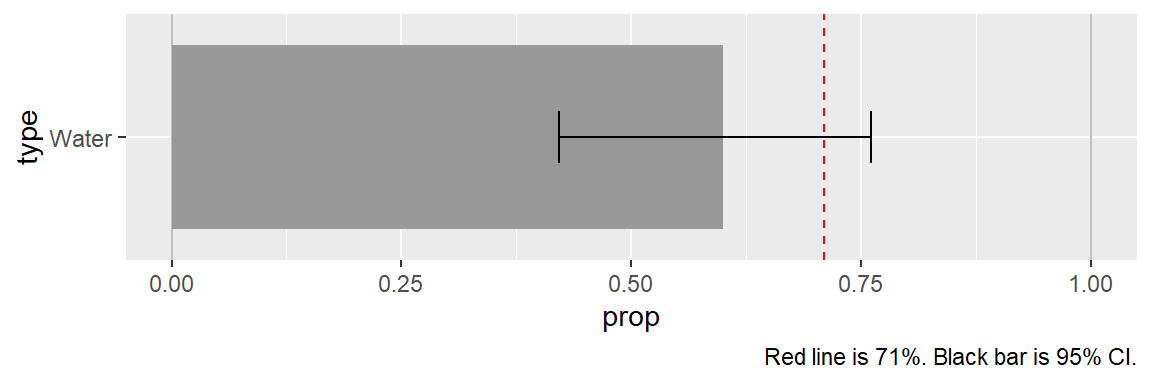Hypothesis test

1. ’’

• $H_0:$ versus $H_A:$
2. Let $$\alpha=0.05$$, the significance level of the test and the Type-I error probability if the null hypothesis is true.

3. $z =$.

4. $p =$ (p-value), this is the observed significance of the test.

5. Because $p =$ (p-value), …

• Also, given your conclusion, state whether you could have made a Type-I or Type-II error and why it is one but not the other.

# African countries in the UN example

In previous years we conducted the following experiment; we will look at Fall 2019, where the priming effect was the weakest.

Previously in class we collected data using a randomized experiment. We provided a priming number (X = 10 or 65, not actually a random number) then asked you two questions:

1. Do you think the percentage of countries represented in the United Nations that are from Africa is higher or lower than X?

2. Give your best estimate of the percentage of countries represented in the United Nations that are from Africa.

The data were compiled into a google doc which we read in below as a csv file.

# UN Africa survey
dat_UN_Africa <-
na.omit() %>%
mutate(
PrimingNumber = factor(PrimingNumber)
, HighLow       = factor(HighLow)
) %>%
filter(
Class == "F19"
)
Rows: 198 Columns: 4
── Column specification ────────────────────────────────────────────────────────
Delimiter: ","
chr (2): HighLow, Class
dbl (2): PrimingNumber, UN_Percentage

ℹ Use spec() to retrieve the full column specification for this data.
ℹ Specify the column types or set show_col_types = FALSE to quiet this message.
str(dat_UN_Africa)
tibble [69 × 4] (S3: tbl_df/tbl/data.frame)
$PrimingNumber: Factor w/ 2 levels "10","65": 2 2 2 2 1 1 1 1 1 2 ...$ HighLow      : Factor w/ 2 levels "H","L": 2 2 2 2 1 1 1 1 1 2 ...
$UN_Percentage: num [1:69] 3 25 30 34 28 15 26 14 35 20 ...$ Class        : chr [1:69] "F19" "F19" "F19" "F19" ...
- attr(*, "na.action")= 'omit' Named int [1:5] 15 16 17 31 32
..- attr(*, "names")= chr [1:5] "15" "16" "17" "31" ...

Here are some summaries and plots.

## If we create a summary data.frame with a similar structure as our data, then we
##   can annotate our plot with those summaries.

mean_UN_Africa <-
dat_UN_Africa %>%
group_by(
PrimingNumber
) %>%
summarize(
UN_Percentage = mean(UN_Percentage)
, .groups = "drop_last"
) %>%
ungroup()

# histogram using ggplot
p1 <- ggplot(dat_UN_Africa, aes(x = UN_Percentage))
p1 <- p1 + geom_histogram(binwidth = 4)
p1 <- p1 + geom_rug()
p1 <- p1 + geom_vline(data = mean_UN_Africa, aes(xintercept = UN_Percentage), colour = "red")
p1 <- p1 + facet_grid(PrimingNumber ~ .)
print(p1)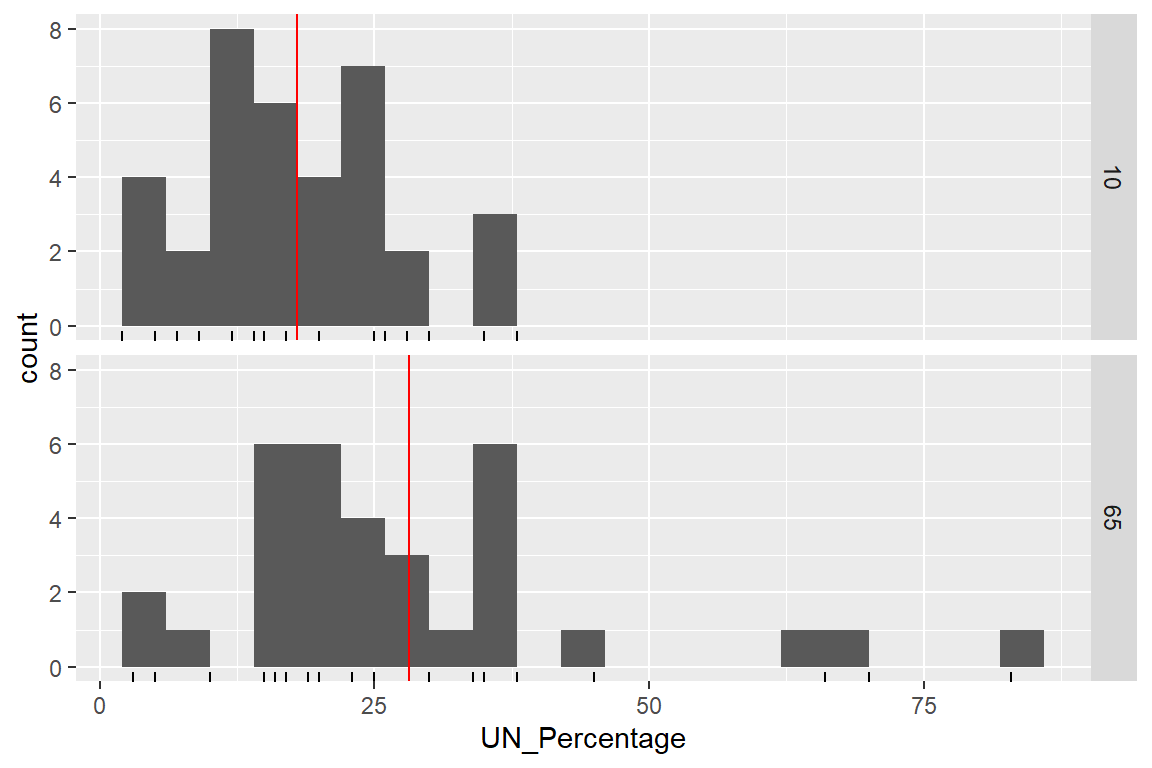# p2 <- ggplot(dat_UN_Africa, aes(x = UN_Percentage, fill=PrimingNumber))
# p2 <- p2 + geom_histogram(binwidth = 4, alpha = 0.5, position="identity")
# p2 <- p2 + geom_rug()
# p2 <- p2 + geom_vline(data = mean_UN_Africa, aes(xintercept = UN_Percentage, colour = PrimingNumber, linetype = PrimingNumber))
# p2 <- p2 + geom_rug(aes(colour = PrimingNumber), alpha = 1/2)
# #print(p2)
#
# # grid.arrange() is a way to arrange several ggplot objects
# library(grid)
# grid.arrange(
#     grobs = list(p1, p2)
#   , ncol = 1
#   )

A priori, before we observed the data, we hypothesized that those who were primed with a larger number (65) would provide a higher percentage (UN_Percentage) than those with the lower number (10). Therefore, this is a one-sided test.

1. (4 p) Set up the two-sample t-test and state the conclusions.
# two-sample t-test
t_summary_UN <-
t.test(
UN_Percentage ~ PrimingNumber
, data = dat_UN_Africa
, alternative = "less"
)

t_summary_UN

Welch Two Sample t-test

data:  UN_Percentage by PrimingNumber
t = -2.9877, df = 47.438, p-value = 0.00222
alternative hypothesis: true difference in means between group 10 and group 65 is less than 0
95 percent confidence interval:
-Inf -4.463638
sample estimates:
mean in group 10 mean in group 65
17.97222         28.15152 
e_plot_ttest_pval(t_summary_UN)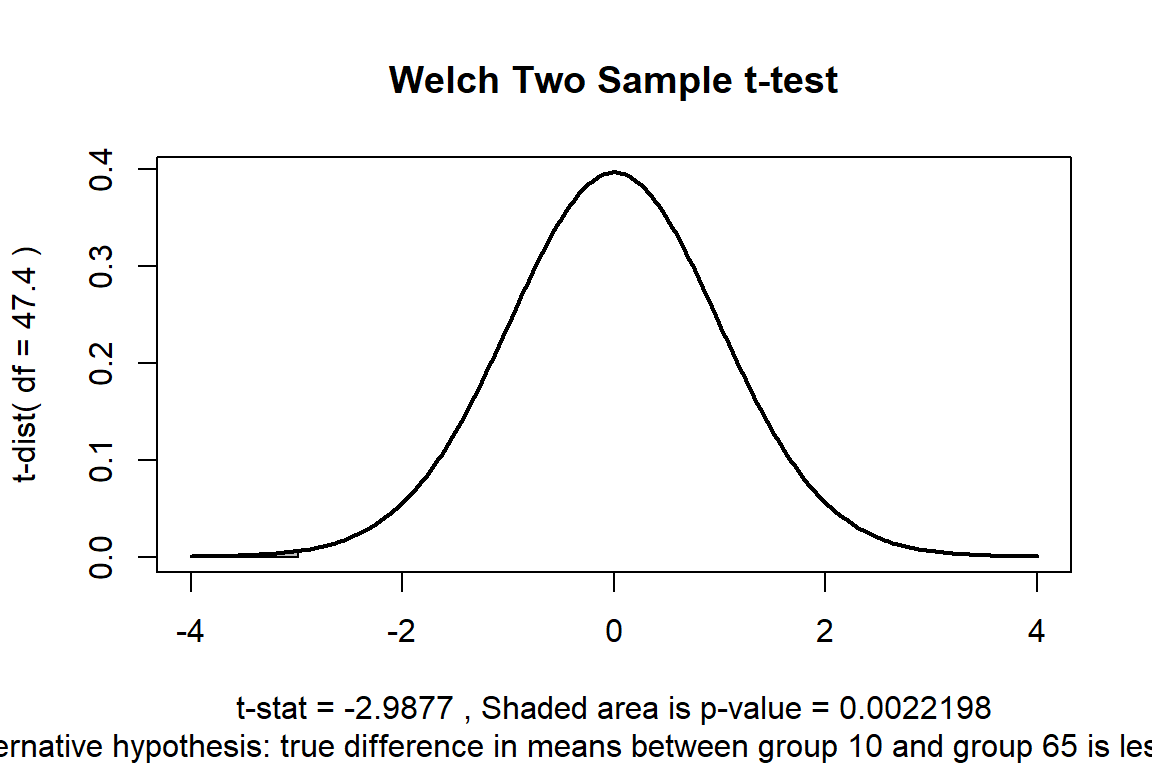Hypothesis test

1. ’’

• $H_0:$ versus $H_A:$
2. Let $$\alpha=0.05$$, the significance level of the test and the Type-I error probability if the null hypothesis is true.

3. $t_{s} =$.

4. $p =$, this is the observed significance of the test.

5. Because $p =$, …

• Also, given your conclusion, state whether you could have made a Type-I or Type-II error and why it is one but not the other.